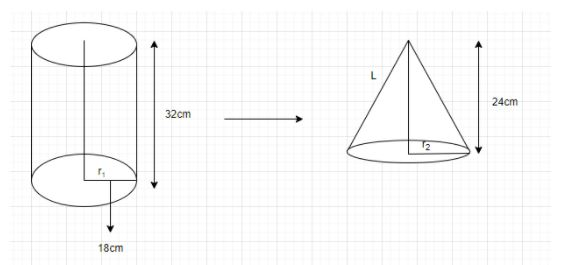QuestionAnswers

# A girl empties a cylindrical bucket, full of sand, of base radius 18 cm and height 32 cm on the floor to form a conical heap of sand. If the height of this conical heap is 24 cm, then find its slant height correct up to one place of decimal.Hint: Here we will proceed from calculating the volume of sand by using the formula of volume of cylinder i.e. $\pi {r^2}h$. Also we will use a formula of volume of conical heap i.e. $= \dfrac{1}{3}\pi {r_2}^2h$ to calculate the volume of the cylindrical bucket.

Now,Let ${r_1}$ and ${r_2}$ be the radius of cylinder and conical heap respectively.
Now we know that volume of cylinder $= \pi {r_1}^2h$
And volume of conical heap $= \dfrac{1}{3}\pi {r_2}^2h$
$\Rightarrow$ Volume of bucket full of sand will be $\pi {r_1}^2h$
$\Rightarrow$ Volume of cone $= \dfrac{1}{3}\pi {r_2}^2h$
At first we will find volume of sand using the below mentioned formula-
$\Rightarrow$ $\pi {r_1}^2h$
$= \dfrac{{22}}{7} \times 18 \times 18 \times 32$
= 32585.1429------(i)
Also we know that cylindrical buckets are emptied into conical heaps so both will have the same amount of sand.
Now we will find the volume of the cylindrical bucket by using the formula of volume of conical heap.
Therefore , Volume of conical heap $= \dfrac{1}{3}\pi {r_2}^2h$------(ii)
Comparing equation (i) and (ii) we get,
Or $\dfrac{1}{3} \times \dfrac{{22}}{7} \times {r_2}^2 \times h = 32585.1429$
Now we calculate the value of $r_2^2$
$\Rightarrow $r_2^2 = 32585.1429 \times 7 \times$\dfrac{1}{{22}} \times \dfrac{1}{8}$
Or $r_2^2 = \sqrt {1296}$
$\Rightarrow$radius of conical heap = 36
$\Rightarrow$(Slant height) L = $\sqrt {r_2^2 + {h^2}}$
$\Rightarrow $\sqrt {1296 + 576} \Rightarrow$\sqrt {1872}$
$\Rightarrow$L = 43.26
Since we have to find the value of slant height of conical heap to one place of decimal, the answer will be 43.3 cm.

Note: While solving this question, one can get confused with the radius of both the shapes because the radius of the conical heap will be different from the radius of the cylindrical bucket. Also we must understand that the slant height of the conical heap will be calculated through a new radius drawn from the volume of the cylindrical bucket. Hence we will get the desired result.

View Notes
Area of a Sector of a Circle FormulaVolume of a Triangular Prism FormulaArea of a Rhombus FormulaPerimeter of a Trapezoid FormulaElectric Field of a SphereSummary of a Photograph - PoemCentroid of a Trapezoid FormulaReconstitution of a Partnership FirmHalf-Life of a ReactionThe Making of a ScientistCBSE Class 10 Hindi A Question Paper 2020Hindi A Class 10 CBSE Question Paper 2009Hindi A Class 10 CBSE Question Paper 2015Hindi A Class 10 CBSE Question Paper 2016Hindi A Class 10 CBSE Question Paper 2012Hindi A Class 10 CBSE Question Paper 2010Hindi A Class 10 CBSE Question Paper 2007Hindi A Class 10 CBSE Question Paper 2013Hindi A Class 10 CBSE Question Paper 2008Hindi A Class 10 CBSE Question Paper 2014RS Aggarwal Class 8 Mathematics Solutions for Chapter-18 Area of a Trapezium and a PolygonNCERT Solutions for Class 12 Accountancy Chapter 4 - Reconstitution of a Partnership Firm:Retirement/Death of a PartnerNCERT Solutions for Class 12 Accountancy Chapter 3 - Reconstitution of a Partnership Firm: Admission of a PartnerRD Sharma Solutions for Class 9 Maths Chapter 19 - Surface Area and Volume of A Right Circular CylinderNCERT Solutions for Class 10 English Footprints Without Feet Chapter 4 - A Question of TrustRD Sharma Solutions for Class 9 Maths Chapter 21 - Surface Area and Volume of a SphereRS Aggarwal Solutions Class 8 Chapter-18 Area of a Trapezium and a Polygon (Ex 18B) Exercise 18.2NCERT Solutions for Class 10 English Footprints Without Feet Chapter 1 - A Triumph of SurgeryNCERT Solutions of Class 6 English Chapter 1 - A Tale of Two BirdsRS Aggarwal Solutions Class 8 Chapter-18 Area of a Trapezium and a Polygon (Ex 18C) Exercise 18.3